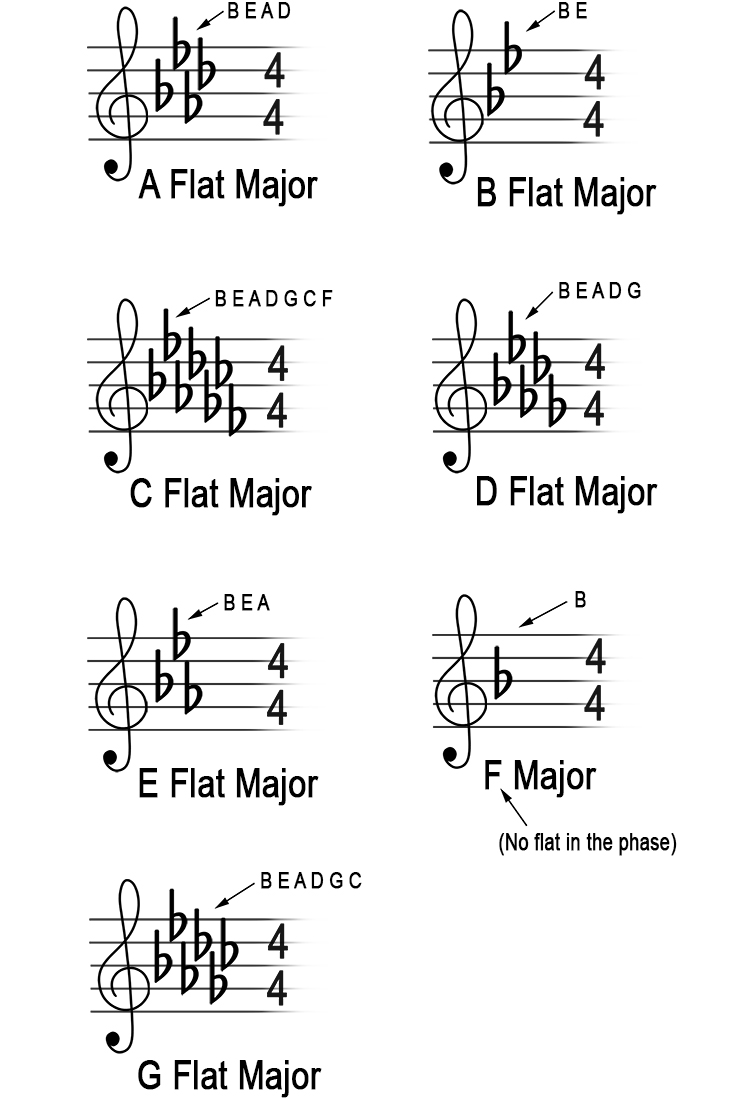# Given the major key, what are the flats?

The order of the flats are always
B E A D G C F

The above is recalled by remembering: 'Fat Cats Go Down Alleys Eating Bacon' but they are the opposite way round.

We know the order of the flats but if you are asked in an exam what are the flats in D flat major, this is how you would work it out:

The letter given is the second flat key from the right.
Use every sharp letter plus one more in the order of the flat key signature.

If the major sharp key listed is:

A flat major (then it is up to D). Flats = B E A D

B flat major (then it is up to E). Flats = B E

C flat major (then it is up to F). Flats = B E A D G C F

D flat major (then it is up to G). Flats = B E A D G

E flat major (then it is up to A). Flats = B E A

F major (then it is up to B).        Flats = B

G flat major (then it is up to C). Flats = B E A D G C